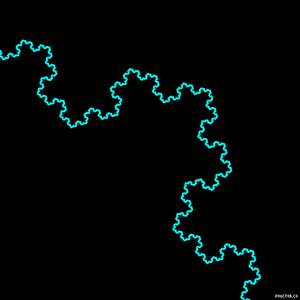## DEV Community

Dan Spratling

Posted on • Updated on • Originally published at danspratling.dev

# Understand recursion in 2 minutes

Whether you've been in programming for a long time, or just starting out, you'll have heard of recursion, but it can be hard to wrap your head around.

Recursion is the act of something repeating itself until an escape is found.Let's create a function which we can use recursively. I'm going to create a function which allows us to multiply a number exponentially - multiplying a number by itself.

``````const multiplyExponential = (number, power) => {
return index !== 0
? number * multiplyExponential(number, index - 1)
: 1
}
``````

Recursive functions don't have to be complicated, though they can be harder to write as it's a different way of thinking. So what's going on?

``````// create a function and pass in the number we want to multiply, and how many times we want to multiply it.
const multiplyExponential = (number, power) => {
return power !== 0
// if the calculation hasn't completed, we return the number multiplied by the value returned from the function
? number * multiplyExponential(number, power - 1)
//if we're at the end, we just want to multiply by 1 so nothing changes
: 1
}

multiplyExponential(7, 4)
// 7 * 7 * 7 * 7 * 1
//<- 2401
``````

Warning! When writing code you don't want to create an infinite loop, you need a way to stop eventually. Make sure you always have a way to escape.

Recursive functions have a fancy name but aren't too different from regular functions. The key difference is that it is a function which calls itself - and that's it!

Mathematics is a great place to start learning about recursive functions but they can be used in other areas of web development too. I've used recursive components to create navigation menus with nested menus.